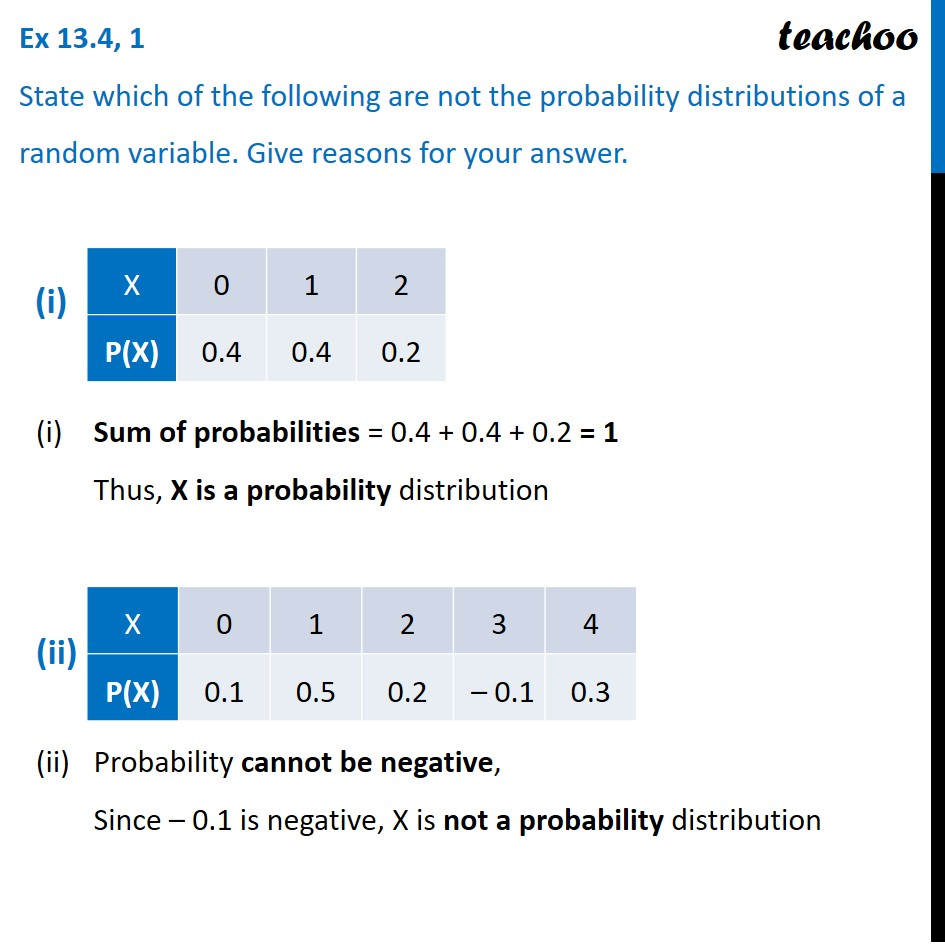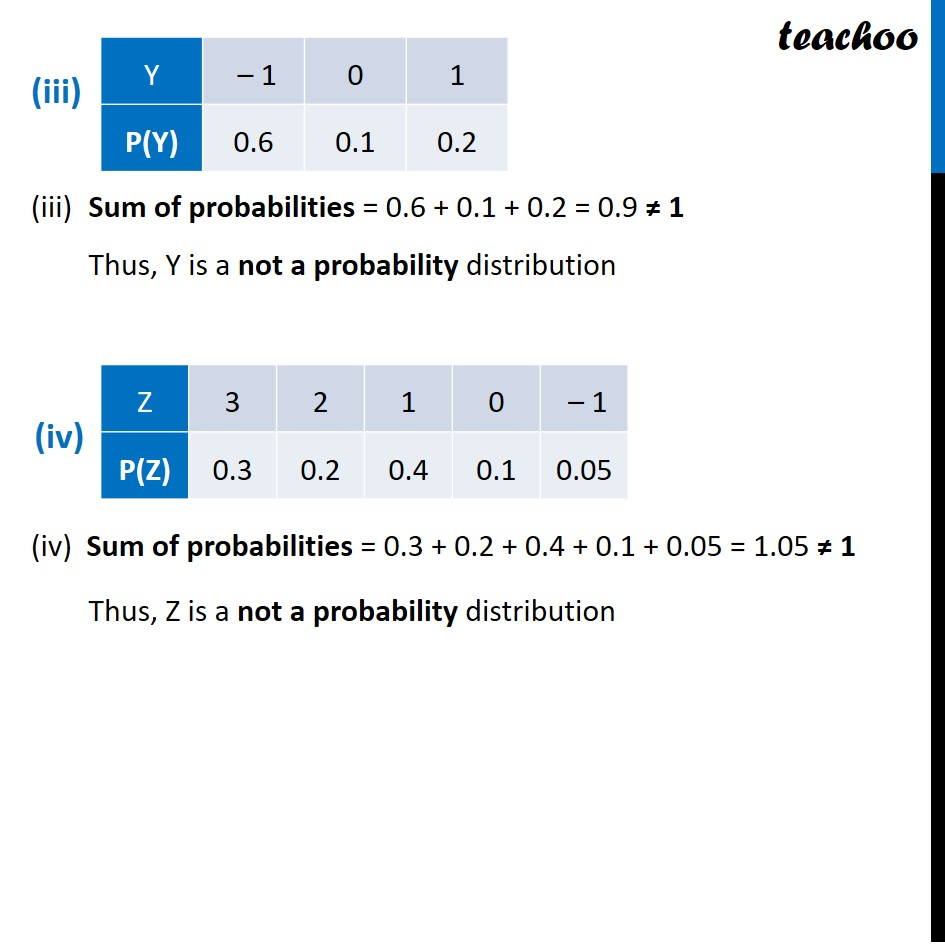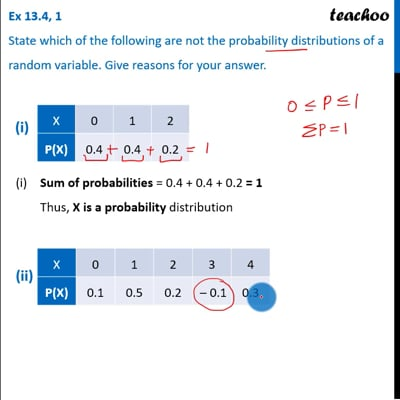Ex 13.4

Chapter 13 Class 12 Probability
Serial order wiseThis video is only available for Teachoo black users

Introducing your new favourite teacher - Teachoo Black, at only ₹83 per month

### Transcript

Ex 13.4, 1 State which of the following are not the probability distributions of a random variable. Give reasons for your answer. (i) Sum of probabilities = 0.4 + 0.4 + 0.2 = 1 Thus, X is a probability distribution (ii) Probability cannot be negative, Since – 0.1 is negative, X is not a probability distribution (iii) Sum of probabilities = 0.6 + 0.1 + 0.2 = 0.9 ≠ 1 Thus, Y is a not a probability distribution (iv) Sum of probabilities = 0.3 + 0.2 + 0.4 + 0.1 + 0.05 = 1.05 ≠ 1 Thus, Z is a not a probability distribution